Chapter 1

Complex Behavior of Simple Systems

Julien Clinton Sprott

Department of Physics

sprott@juno.physics.wisc.edu

1.1. Introduction

Since the seminal work of Lorenz  and Rössler , it has been known that complex behavior (chaos) can occur in systems of autonomous ordinary differential equations (ODEs) with as few as three variables and one or two quadratic nonlinearities. Many other simple chaotic systems have been discovered and studied over the years, but it is not known whether the algebraically simplest chaotic flow has been identified. For continuous flows, the Poincaré-Bendixson theorem [Hirsch 1974] implies the necessity of three variables, and chaos requires at least one nonlinearity. With the growing availability of powerful computers, many other examples of chaos have been subsequently discovered in algebraically simple ODEs. Yet the sufficient conditions for chaos in a system of ODEs remain unknown.

This paper will review the history of recent attempts to identify the simplest such system and will describe two candidate systems that are simpler than any previously known. They were discovered by a brute-force numerical search for the algebraically simplest chaotic flows. There are reasons to believe that these cases are the simplest examples with quadratic and piecewise linear nonlinearities. The properties of these systems will be described.

1.2. Lorenz and Rössler Systems

The celebrated Lorenz equations are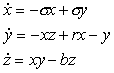(1)
Note that there are seven terms on the right-hand side of these equations, two of which are nonlinear. Also note that there are three parameters. The other four coefficients can be set to unity without loss of generality since x, y, z, and t can be arbitrarily rescaled.

Although the Lorenz system is often taken as the prototypical autonomous dissipative chaotic flow, it is less simple than the Rössler system given by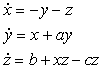(2)
which also has seven terms and three parameters, but only a single quadratic nonlinearity.

Other autonomous chaotic flows that are algebraically simpler than Eq. (2) have also been discovered. For example, Rössler  found chaos in the system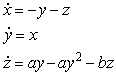(3)
which has a single quadratic nonlinearity but only six terms and two parameters.

More recently, we embarked on an extensive computer search for chaotic systems with five terms and two quadratic nonlinearities or six terms and one quadratic nonlinearity [Sprott 1994]. We found five cases of the former type and fourteen of the latter type. One of these cases was conservative and previously known [Posch 1986], and the others were dissipative and apparently previously unknown.

In response to this work, Gottlieb  pointed out that one of our examples can be recast into the explicit third-order scalar form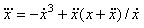(4)
which he called a "jerk function" since it involves a third derivative of x, which in a mechanical system is the rate of change of acceleration, sometimes called a "jerk" [Schot 1978]. Gottlieb asked the provocative question, "What is the simplest jerk function that gives chaos?"

In response to this question, Linz  showed that the Lorenz and Rössler models have relatively complicated jerk representations, but that one of our examples can be written as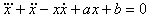(5)
In a subsequent paper [Eichhorn 1998], Linz and coworkers showed that all of our cases with a single nonlinearity and some others could be organized into a hierarchy of quadratic jerk equations with increasingly many terms. They also derived criteria for functional forms of the jerk function that cannot exhibit chaos.

We also took up Gottliebs challenge and discovered a particularly simple case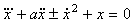(6)
which has only a single quadratic nonlinearity and a single parameter [Sprott 1997]. With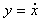and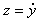, this three-dimensional dynamical system has only five terms. It exhibits chaos for a = 2.017 with an attractor as shown in Fig. 1. For this value of a, the Lyapunov exponents (base-e) are (0.0550, 0, -2.0720) and the Kaplan-Yorke dimension is DKY = 2.0265. It is unlikely that a simpler quadratic form exists because it would have no adjustable parameters. The number of possibilities is quite small, and a systematic numerical check revealed that none of them exhibits chaos. Furthermore, Fu  and Heidel  proved that all three-dimensional dynamical systems with quadratic nonlinearities and fewer than five terms cannot exhibit chaos.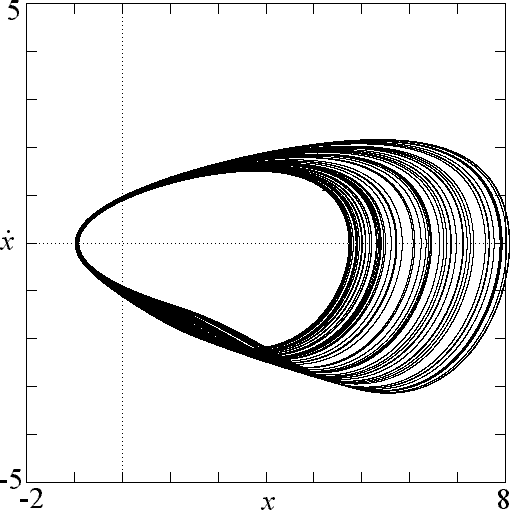Figure 1. Attractor for the simplest chaotic flow with a quadratic nonlinearity from Eq. (6) with a = 2.017.

This system and most of the other cases that we found share a common route to chaos. The control parameter a can be considered a damping rate for the nonlinear oscillator. For large values of a, there is one or more stable equilibrium points. As a decreases, a Hopf bifurcation occurs in which the equilibrium becomes unstable, and a stable limit cycle is born. The limit cycle grows in size until it bifurcates into a more complicated limit cycle with two loops, which then bifurcates into four loops, and so forth, in a sequence of period doublings, until chaos finally onsets. A further decrease in a causes the chaotic attractor to grow in size, passing through infinitely many periodic windows, and finally becoming unbounded when the attractor grows to touch the boundary of its basin of attraction (a crisis). A bifurcation diagram for Eq. (6) is shown in Fig. 2. In this figure, the local maxima of x are plotted as the damping a is gradually decreased. Note that the scales are plotted backwards to emphasize the similarity to the logistic map. Indeed, a plot of the maximum x versus the previous maximum in Fig. 3 shows an approximate parabolic dependence, albeit with a very small-scale fractal structure.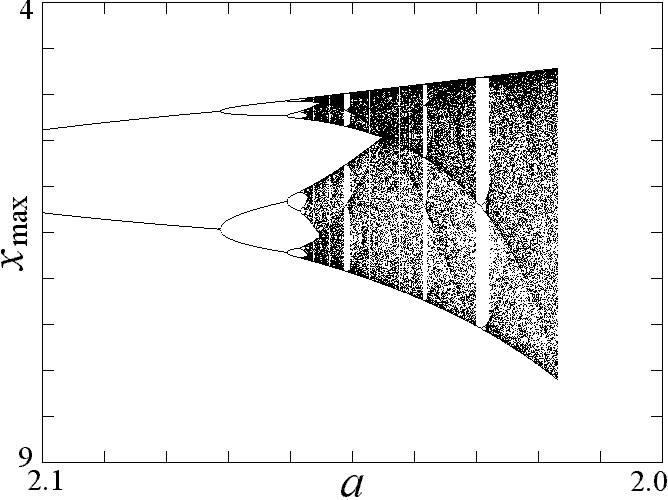Figure 2. Bifurcation diagram for Eq. (6) as the damping is reduced.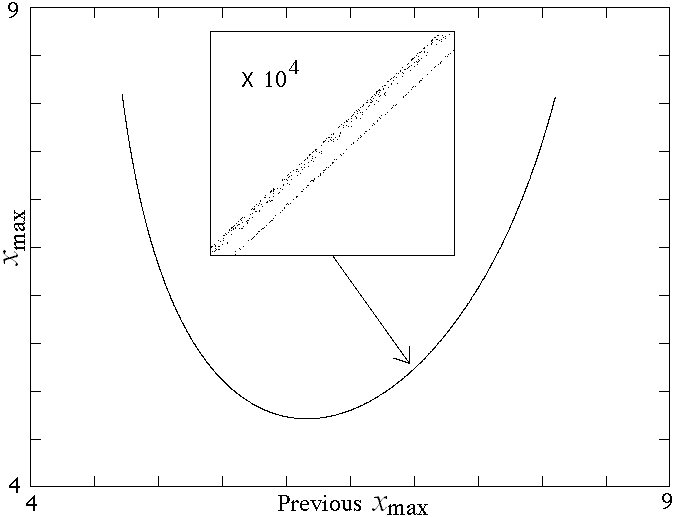Figure 3. Return map showing each value of xmax versus the previous value of xmax for Eq. (6) with a = 2.017. The insert shows fractal structure at a magnification of 104.

1.4. Piecewise Linear Jerk Systems

Having found what appears to be the simplest jerk function with a quadratic nonlinearity that leads to chaos, it is natural to ask whether the nonlinearity can be weakened. In particular, the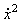term in Eq. (6) might be replaced with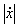. A numerical search did not reveal any such chaotic solutions. However, the system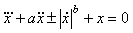(7)
which is equivalent to Eq. (6) for b = 2, has chaotic solutions for 1 < b < 3 as shown in Fig. 4, although the size of the attractor scales approximately at exp[1/(b-1)] and becomes infinite as b approaches 1. The spiral structure of the chaotic region in a-b space begs for an explanation.Figure 4. Regions of a-b space for which chaos occurs in Eq. (7).

In an extensive numerical search for the algebraically simplest dissipative chaotic flow with an absolute-value nonlinearity, Linz and Sprott [Linz, 1999] discovered the case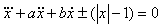(8)
which exhibits chaos for a = 0.6 and b = 1 with an attractor as shown in Fig. 5. For these parameters, the Lyapunov exponents (base-e) are (0.035, 0, -0.635), and the Kaplan-Yorke dimension is DKY = 2.055. The constant 1 affects only the size of the attractor. Chaos exists for arbitrarily small values of this constant, but the attractor and its basin of attraction shrink proportionally. Chaos also occurs in Eq. (8) with |x| replaced by |x|n for any nonzero n.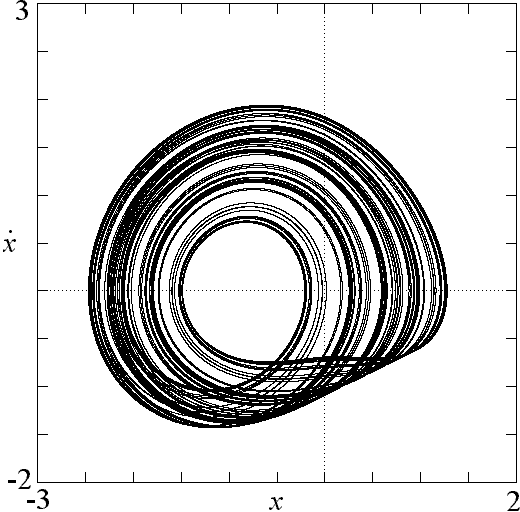Figure 5. Attractor for the simplest chaotic system with an absolute-value nonlinearity from Eq. (8) with a = 0.6 and b = 1.

This system also exhibits a period-doubling route to chaos as shown in Fig. 6 and otherwise resembles the simple quadratic case previously described. This example relates to the quadratic flows as the tent map does to the logistic map. We claim it is the most elementary piecewise linear chaotic flow. Linz  has recently proved that chaos cannot exist in Eq. (8) if any of the terms are set to zero. Furthermore, the piecewise linear nature of the nonlinearity allows for an analytic solution to Eq. (8) by solving two linear equations and matching the boundary conditions at x = 0 [Linz 1999].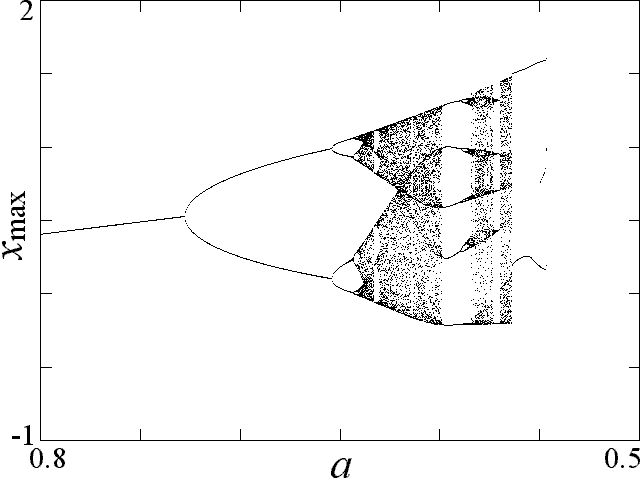Figure 6. Bifurcation diagram for Eq. (8) with b = 1 as the damping is reduced.

Equation (8) is a special case of the more general system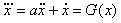(9)
in which G(x) is any one of a large number of nonlinear functions [Sprott 2000]. Integration of Eq. (9) shows that it is a damped harmonic oscillator driven by a nonlinear memory term that depends on its positional history. Such an equation often arises in the feedback control of an oscillator in which the experimentally accessible variable is a transformed and integrated version of the fundamental dynamical variable. Despite its importance and the richness of its dynamics, this system has been relatively little studied [Coulett 1979].

1.5. Electrical Circuit Implementations

Piecewise linear forms of G(x) lend themselves to electronic implementation using diodes and operational amplifiers [Sprott 2000]. One example of such a circuit that solves Eq. (8) electronically is shown in Fig. 7. In this circuit, all the capacitors are 0.1 microfarads, and the resistors are 1 kilo-ohms except for the variable resistor, whose value is the inverse of the damping constant a in Eq. (8) in units of kilo-ohms. The non-inverting inputs to the amplifiers are grounded and not shown. The fundamental frequency at the onset of oscillation is 1592 Hz (5000/pi). The period doublings, periodic windows, and chaos are easily made audible by connecting the output x to an amplifier and speaker. Such circuits are similar in spirit to Chuas circuit [Matsumoto 1985] but are easier to implement and analyze.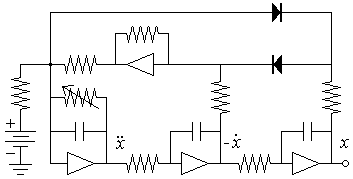Figure 7. A chaotic circuit using inverting operational amplifiers and diodes that solves Eq. (8).

1.6. Conclusions

Two new dissipative chaotic systems, given by Eq. (6) and Eq. (8), have been described that are algebraically simpler than the Lorenz and Rössler attractors. One has a quadratic nonlinearity, and the other has an absolute value nonlinearity. Each system is apparently the algebraically simplest dissipative chaotic system of its type. The latter case is especially suited for electronic implementation using diodes and operational amplifiers.

References

Coullet, P., Tresser, C., & Arneodo, A., 1979, A Transition to Stochasticity for a Class of Forced Oscillators. Phys. Lett. A, 72, 268.

Eichhorn, R., Linz, S.J., & Hänggi, P., 1998, Transformations of Nonlinear Dynamical Systems to Jerky Motion and its Application to Minimal Chaotic Flows. Phys. Rev. E, 58, 7151.

Fu, Z., & Heidel, J., 1997, Non-Chaotic Behavior in Three-Dimensional Quadratic Systems. Nonlinearity, 10, 1289.

Gottlieb, H.P.W., 1996, What is the Simplest Jerk Function that gives Chaos? Am. J. Phys., 64, 525.

Heidel, J., & Fu, Z., 1999, Nonchaotic Behavior in Three-Dimensional Quadratic Systems II. The Conservative Case. Nonlinearity, 12, 617.

Hirsch, H.W., & Smale, S., 1974, Differential Equations, Dynamical Syustems and Linear Algebra, Academic Press (New York), 11, 239.

Linz, S.J., 1997, Nonlinear Dynamical Models and Jerky Motion. Am. J. Phys., 65, 523.

Linz, S.J., & Sprott, J.C., 1999, Elementary Chaotic Flow. Phys. Lett. A, 259, 240.

Linz, S.J., 2000, No-chaos Criteria for Certain Jerky Dynamics. submitted for publication.

Lorenz, E.N., 1963, Deterministic Nonperiodic Flow. J. Atmos. Sci., 20, 130.

Matsumoto, T., Chua, L.O., & Komoro, M., The Double Scroll. IEEE Trans. Circuits Syst., CAS-32, 797.

Posh, H.A., Hoover, W.G., & Vesely, F.J., 1986, Canonical Dynamics of the Nosé Oscillator: Stability, Order, and Chaos. Phys. Rev. A, 33, 4253.

Rössler, O.E., 1976, An Equation for Continuous Chaos. Phys. Lett. A, 57, 397.

Rössler, O.E., 1979, Continuous Chaos  Four Prototype Equations. Am. (N.Y.) Acad. Sci., 316, 376.

Schot, S.H., 1978, The Time Rate of Change of Acceleration. Am. J. Phys., 46, 1090.

Sprott, J.C., 1994, Some Simple Chaotic Flows. Phys. Rev. E, 50, R647.

Sprott, J.C., 1997, Simplest Dissipative Chaotic Flow. Phys. Lett. A, 228, 271.

Sprott, J.C., 2000, A New Class of Chaotic Circuit. Phys. Lett A, 266, 19.